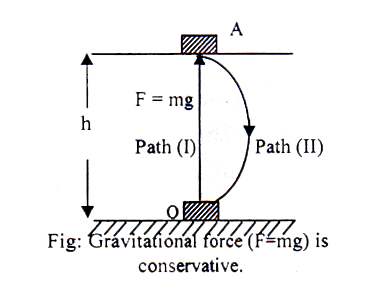# Conservative and non-Conservative force

Conservative force: A force is said to be conservative if the work done by the force in moving a body along a closed path is zero. This force depends only on the initial and final position of the body, and not on the nature of path followed between the initial and final positions. Gravitational force is a conservative force.Explanation: Let us consider a body of mass m is at point O at the ground, to raise the body at a height h at point A through path-I, the work done against the force of gravity is,

WOA = -mgh ; [ mg = F = Force of gravity]

If the body is allowed to fall from O through path-II, as shown in figure, the work done by the force of gravity is,

WAO = mgh

So, The total work done for the (closed path) round trip is,

WOA + WAO = -mgh + mgh = mg (- h + h) = F (- h + h) =0

The total work done is zero by the gravitational force. Hence it is a conservative force.

Non-Conservative force: If the work done by a force in moving a body along a closed path is non-zero, the force is said to be a non-conservative. This force depends on the path followed between the initial and final positions of the body. The frictional force is non-conservative.